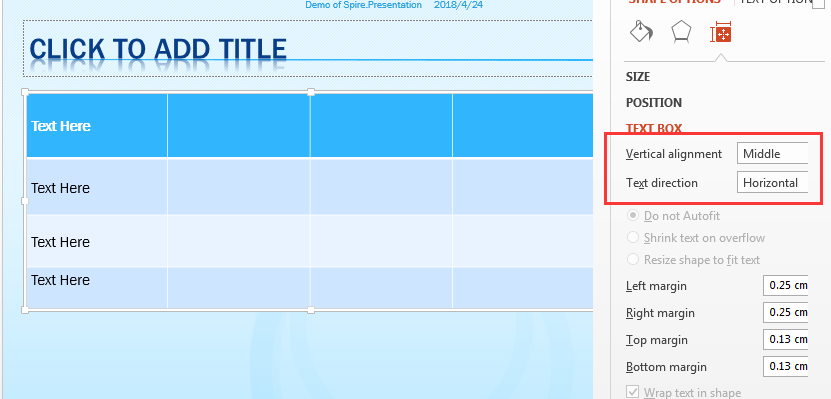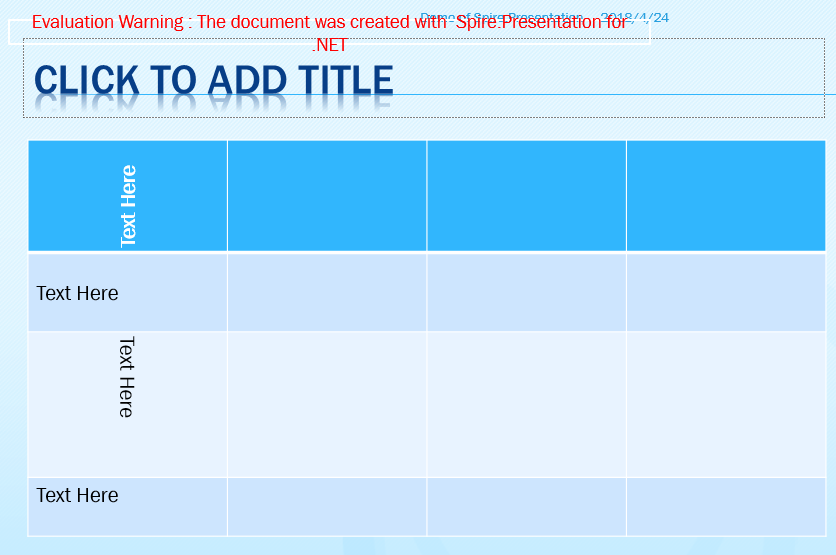# Vertically align the text in table cell on Presentation slides

Spire.Presentation supports to align text vertically in a table cell on the presentation slides. In this article, we will show you how to use C# to align the text in table cell and set the text direction. Firstly, view the screenshot on Microsoft PowerPoint of the vertical alignment and the text direction:Detail steps:

Step 1: Create a new PowerPoint document and load the sample document from file.

```Presentation ppt = new Presentation();
```

Step 2: Get the table shape from the first presentation slide.

```ITable table = null;
foreach (IShape shape in ppt.Slides.Shapes)
{
if (shape is ITable)
{
table = (ITable)shape;
```

Step 3: Aligning the text vertically and set the text direction.

```table[i, 0].TextAnchorType = TextAnchorType.Center;
table[i, 0].VerticalTextType = VerticalTextType.Vertical270;

table[i, 1].TextAnchorType = TextAnchorType.Center;
table[i, 1].VerticalTextType = VerticalTextType.Horizontal;

table[i, 2].TextAnchorType = TextAnchorType.Center;
table[i, 2].VerticalTextType = VerticalTextType.Vertical;
```

Step 4: Save the document to file.

```ppt.SaveToFile("Result.pptx", FileFormat.Pptx2010);
```

Effective screenshot after set the vertical alignment and the text direction:Full codes of vertical align the text and set the text direction.:

```Presentation ppt = new Presentation();

ITable table = null;
foreach (IShape shape in ppt.Slides.Shapes)
{
if (shape is ITable)
{
table = (ITable)shape;

for (int i = 0; i < table.ColumnsList.Count; i++)
{
table[i, 0].TextAnchorType = TextAnchorType.Center;
table[i, 0].VerticalTextType = VerticalTextType.Vertical270;

table[i, 1].TextAnchorType = TextAnchorType.Center;
table[i, 1].VerticalTextType = VerticalTextType.Horizontal;

table[i, 2].TextAnchorType = TextAnchorType.Center;
table[i, 2].VerticalTextType = VerticalTextType.Vertical;

}
}
}

ppt.SaveToFile("Result.pptx", FileFormat.Pptx2010);```